Next: 4.6 Multiple Material Regions Up: 4. A Fast Level Previous: 4.4.3 Pattern Transfer

# 4.5 Smoothing

The LS method also provides a simple way to smooth a given geometry. Setting the surface velocity in the LS equation equal to the mean curvature leads to a smoothed surface . The definition of the mean curvature and its approximation were given in Section 3.3.2. The introduction of a lower limit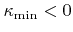and an upper limit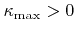for regions with negative and positive curvature, respectively, controls the amount of smoothing. The smoothing algorithm is realized by setting the surface velocity field as follows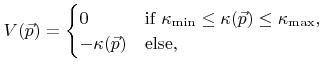(4.17)

and solving the LS equation over time until all surface velocities are equal to 0. Figure 4.7 demonstrates smoothing for the test structure given in Figure 4.5 with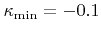and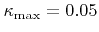.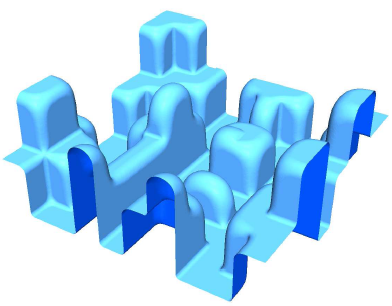Next: 4.6 Multiple Material Regions Up: 4. A Fast Level Previous: 4.4.3 Pattern Transfer

Otmar Ertl: Numerical Methods for Topography Simulation